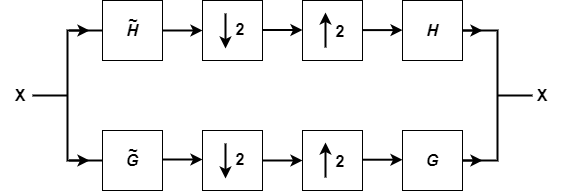# isbiorthwfb

Determine if filter bank is biorthogonal wavelet filter bank

Since R2022b

## Syntax

``tf = isbiorthwfb(LoR,LoD)``
``tf = isbiorthwfb(LoR,LoD,HiR,HiD)``
``tf = isbiorthwfb(___,Tolerance=tol)``
``[tf,checks] = isbiorthwfb(___)``

## Description

````tf = isbiorthwfb(LoR,LoD)` returns true if the two-channel filter bank formed from the lowpass filters `LoR` and `LoD` satisfy the necessary and sufficient conditions to be a two-channel biorthogonal perfect reconstruction (PR) wavelet filter bank. `isbiorthwfb` forms the dual highpass (wavelet) filters using the `qmf` function: `HiD = qmf(LoR)``HiR = qmf(LoD)` For a list of the necessary and sufficient conditions that the lowpass and highpass filters must satisfy, see Biorthogonal Perfect Reconstruction Wavelet Filter Bank.```
````tf = isbiorthwfb(LoR,LoD,HiR,HiD)` uses the four filters `LoR`, `LoD`, `HiR`, and `HiD` to determine whether the four filters jointly satisfy the necessary and sufficient conditions to be a two-channel biorthogonal PR wavelet filter bank.```
````tf = isbiorthwfb(___,Tolerance=tol)` uses the positive real scalar tolerance `tol` to determine whether the filters satisfy the necessary and sufficient conditions to be a two-channel biorthogonal PR wavelet filter bank.```

example

````[tf,checks] = isbiorthwfb(___)` returns a table with all orthogonality checks.```

## Examples

collapse all

Use `wfilters` to obtain the biorthogonal spline wavelet filters with 3 vanishing moments in the analysis filters and 1 vanishing moment in the synthesis filters. The sum of the coefficients in each lowpass filter equals $\sqrt{2}$.

```[LoD,HiD,LoR,HiR] = wfilters("bior3.1"); sum(LoD)-sqrt(2)```
```ans = 2.2204e-16 ```
`sum(LoR)-sqrt(2)`
```ans = 2.2204e-16 ```

Test the biorthogonality of the filters.

`[tf,checks] = isbiorthwfb(LoR,LoD,HiR,HiD)`
```tf = logical 1 ```
```checks=7×3 table Pass-Fail Maximum Error Test Tolerance _________ _____________ ______________ Dual filter lengths correct pass 0 0 Filter sums pass 2.2204e-16 1.4901e-08 Zero lag lowpass dual filter cross-correlation pass 2.2204e-16 1.4901e-08 Zero lag highpass dual filter cross-correlation pass 2.2204e-16 1.4901e-08 Even lag lowpass dual filter cross-correlation pass 0 1.4901e-08 Even lag highpass dual filter cross-correlation pass 0 1.4901e-08 Even lag lowpass-highpass cross-correlation pass 0 1.4901e-08 ```

Use `biorwavf` to obtain just the lowpass analysis and synthesis filters of the same wavelet. The sum of the coefficients in each filter equals 1.

```[df,rf] = biorwavf("bior3.1"); sum(df)```
```ans = 1 ```
`sum(rf)`
```ans = 1 ```

Test the biorthogonality of the filters.

`[tf,checks] = isbiorthwfb(rf,df)`
```tf = logical 1 ```
```checks=7×3 table Pass-Fail Maximum Error Test Tolerance _________ _____________ ______________ Dual filter lengths correct pass 0 0 Filter sums pass 2.2204e-16 1.4901e-08 Zero lag lowpass dual filter cross-correlation pass 2.2204e-16 1.4901e-08 Zero lag highpass dual filter cross-correlation pass 2.2204e-16 1.4901e-08 Even lag lowpass dual filter cross-correlation pass 0 1.4901e-08 Even lag highpass dual filter cross-correlation pass 0 1.4901e-08 Even lag lowpass-highpass cross-correlation pass 0 1.4901e-08 ```

## Input Arguments

collapse all

Lowpass filters, specified as a pair of real-valued vectors. One vector is a lowpass analysis filter, and the other vector is a lowpass synthesis filter. `LoR` and `LoD` are not required to have equal or even length. `isbiorthwfb` equalizes the lengths internally using `biorfilt`. `LoR` and `LoD` should sum to 1 or √2.

Data Types: `single` | `double`

Highpass filters, specified as a pair of real-valued vectors. `isbiorthwfb` assumes that the two pairs of vectors `LoR``HiR` and `LoD``HiD` form dual filter pairs.

Data Types: `single` | `double`

Tolerance used in the filter bank checks, specified as a positive real scalar.

## Output Arguments

collapse all

True or false result, returned as a `1` or `0` of data type `logical`. The `isbiorthwfb` function returns a `1` if the filters satisfy all the conditions listed in Biorthogonal Perfect Reconstruction Wavelet Filter Bank within the specified tolerance.

Biorthogonality checks, returned as a table. The table shows `pass` or `fail` for each check as well as the maximum error and specified test tolerance where applicable. A test tolerance of `0` indicates that the check is a logical pass or fail.

collapse all

### Biorthogonal Perfect Reconstruction Wavelet Filter Bank

The lowpass and highpass analysis filters $\stackrel{˜}{G}$ and $\stackrel{˜}{H},$ respectively, and lowpass and highpass synthesis filters $G$ and $H,$ respectively, form a biorthogonal perfect reconstruction (PR) wavelet filter bank if the filters satisfy certain conditions. This is a diagrammatic representation of the two-channel filter bank.Here, $\stackrel{˜}{G}\left(n\right)={\left(-1\right)}^{n}H\left(1-n\right)$ and $G\left(n\right)={\left(-1\right)}^{n}\stackrel{˜}{H}\left(1-n\right)$.

The filters $\stackrel{˜}{G}$, $\stackrel{˜}{H},$, $G$, and $H,$ form a biorthogonal PR wavelet filter bank if the following conditions are satisfied.

• `Dual filter lengths correct` — The length of $\stackrel{˜}{G}$ equals the length of $H,$, and the length of $G$ equals the length of $\stackrel{˜}{H},$.

• `Filter sums` — For both lowpass filters, the sum of the coefficients equals √2. For both highpass filters, the sum of the coefficients equals 0.

• `Zero lag lowpass dual filter cross-correlation` — The zero lag cross-correlation of the lowpass filter $G$ and its dual equals 1: $\sum _{n}\stackrel{˜}{g}\left(n\right)g\left(n\right)=1$

• `Zero lag highpass dual filter cross-correlation` — The zero lag cross-correlation of the highpass filter $H,$ and its dual equals 1: $\sum _{n}\stackrel{˜}{h}\left(n\right)h\left(n\right)=1$

• `Even lag lowpass dual filter cross-correlation` — The even nonzero lag cross-correlation of the lowpass filter and its dual equals 0: $\sum _{n}\stackrel{˜}{g}\left(n\right)g\left(n+2k\right)=0\text{ }\text{for}\text{\hspace{0.17em}}k\ne 0.$

• `Even lag highpass dual filter cross-correlation` — The even nonzero lag cross-correlation of the highpass filter and its dual equals 0 $\sum _{n}\stackrel{˜}{h}\left(n\right)h\left(n+2k\right)=0\text{ }\text{for}\text{\hspace{0.17em}}k\ne 0.$

• `Even lag lowpass-highpass cross-correlation` — The even lag cross-correlation of the lowpass and dual highpass filters equals 0. Similarly, the even lag cross-correlation of the highpass and dual lowpass filters equals 0: $\sum _{n}\stackrel{˜}{g}\left(n\right)h\left(n+2k\right)=\sum _{n}\stackrel{˜}{h}\left(n\right)g\left(n+2k\right)=\text{\hspace{0.17em}}0$

 Strang, Gilbert, and Truong Nguyen. Wavelets and Filter Banks. Rev. ed. Wellesley, Mass: Wellesley-Cambridge Press, 1997.

 Burrus, C. S., Ramesh A. Gopinath, and Haitao Guo. Introduction to Wavelets and Wavelet Transforms: A Primer. Upper Saddle River, N.J: Prentice Hall, 1998.